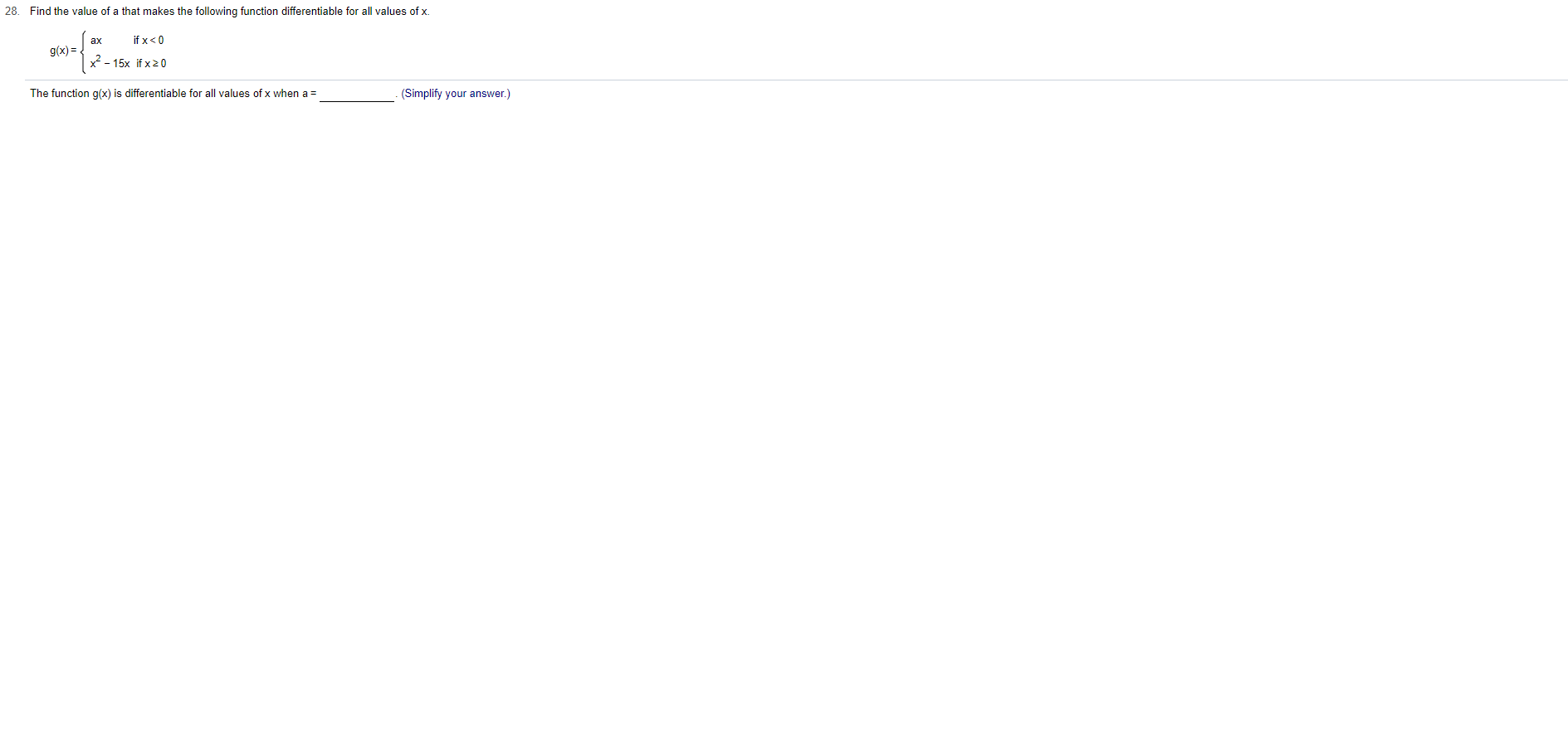# 28.Find the value of a that makes the following function differentiable for all values of x.if x< 0axg(x)=x2-15x if x 20The function g(x) is differentiable for all values of x when a=(Simplify your answer.)

Question
3 views

Can you help me step by step?help_outlineImage Transcriptionclose28. Find the value of a that makes the following function differentiable for all values of x. if x< 0 ax g(x)= x2-15x if x 20 The function g(x) is differentiable for all values of x when a= (Simplify your answer.) fullscreen
check_circle

Step 1

The given function is,

Step 2

Find the left and right hand limits of the...

### Want to see the full answer?

See Solution

#### Want to see this answer and more?

Solutions are written by subject experts who are available 24/7. Questions are typically answered within 1 hour.*

See Solution
*Response times may vary by subject and question.
Tagged in

### Other